# [C描述演算法入門]常見排序小結

theme: channing-cyan highlight: a11y-dark

# 常見排序小結

## 排序的概念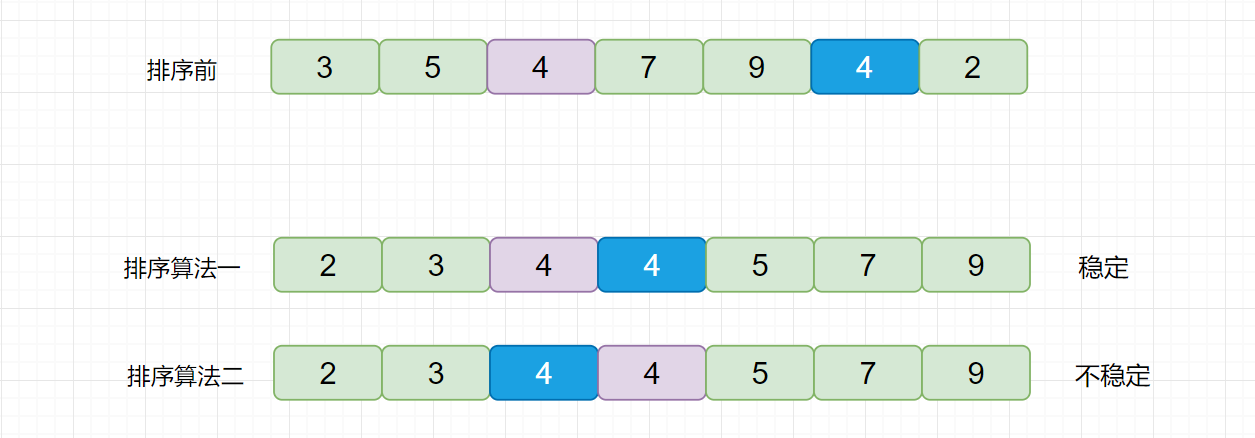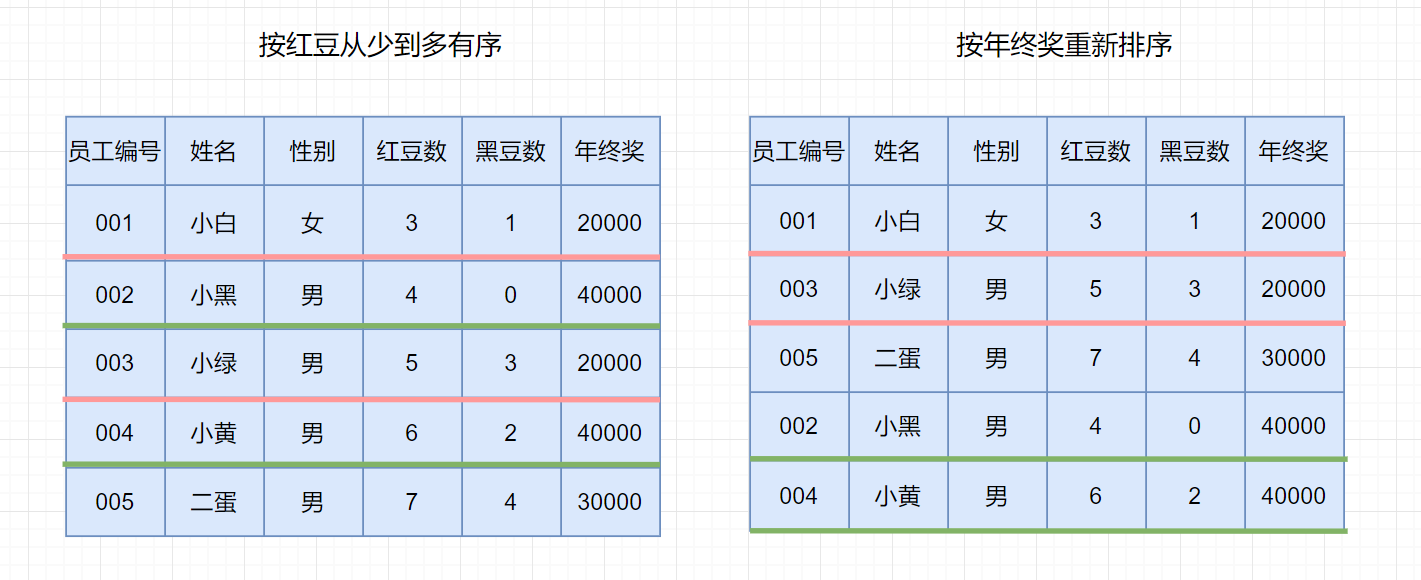## 常見的排序演算法```// 測試排序的效能對比  void TestOP()  {      srand(time(0));      const int N = 100000;      int* a1 = (int*)malloc(sizeof(int)*N);      int* a2 = (int*)malloc(sizeof(int)*N);      int* a3 = (int*)malloc(sizeof(int)*N);      int* a4 = (int*)malloc(sizeof(int)*N);      int* a5 = (int*)malloc(sizeof(int)*N);      int* a6 = (int*)malloc(sizeof(int)*N);            for (int i = 0; i < N; ++i)     {          a1[i] = rand();          a2[i] = a1[i];          a3[i] = a1[i];          a4[i] = a1[i];          a5[i] = a1[i];          a6[i] = a1[i];     }      int begin1 = clock();      InsertSort(a1, N);      int end1 = clock();      int begin2 = clock();      ShellSort(a2, N);      int end2 = clock();      int begin3 = clock();      SelectSort(a3, N);      int end3 = clock();      int begin4 = clock();      HeapSort(a4, N);      int end4 = clock();      int begin5 = clock();      QuickSort(a5, 0, N-1);      int end5 = clock();      int begin6 = clock();      MergeSort(a6, N);      int end6 = clock();            printf("InsertSort:%d\n", end1 - begin1);      printf("ShellSort:%d\n", end2 - begin2);      printf("SelectSort:%d\n", end3 - begin3);      printf("HeapSort:%d\n", end4 - begin4);      printf("QuickSort:%d\n", end5 - begin5);      printf("MergeSort:%d\n", end6 - begin6);            free(a1);      free(a2);      free(a3);      free(a4);      free(a5);      free(a6);  }```

## 排序演算法總結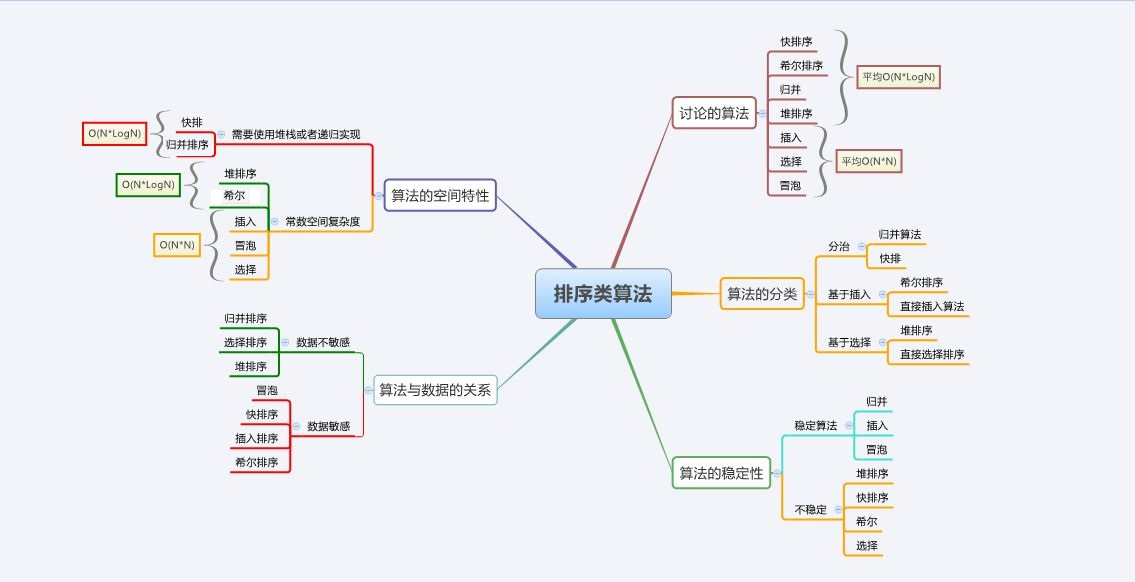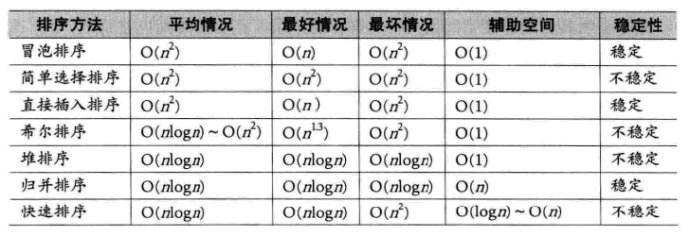### 穩定性分析

#### 氣泡排序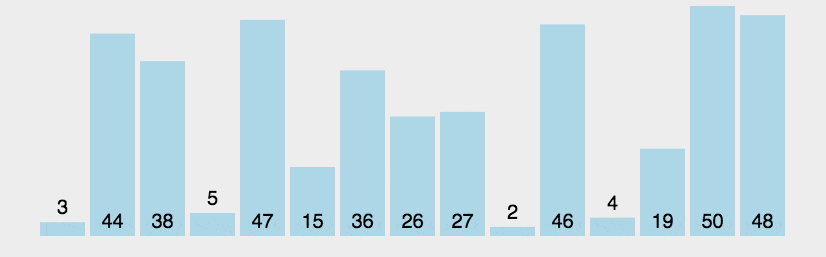#### 直接選擇排序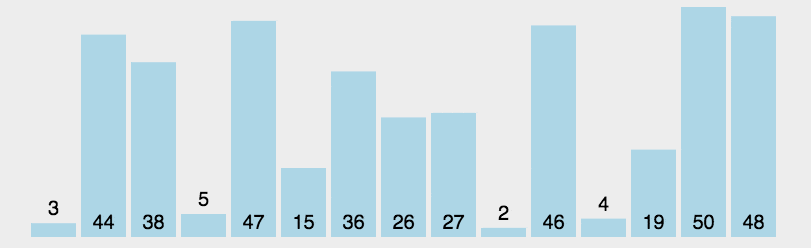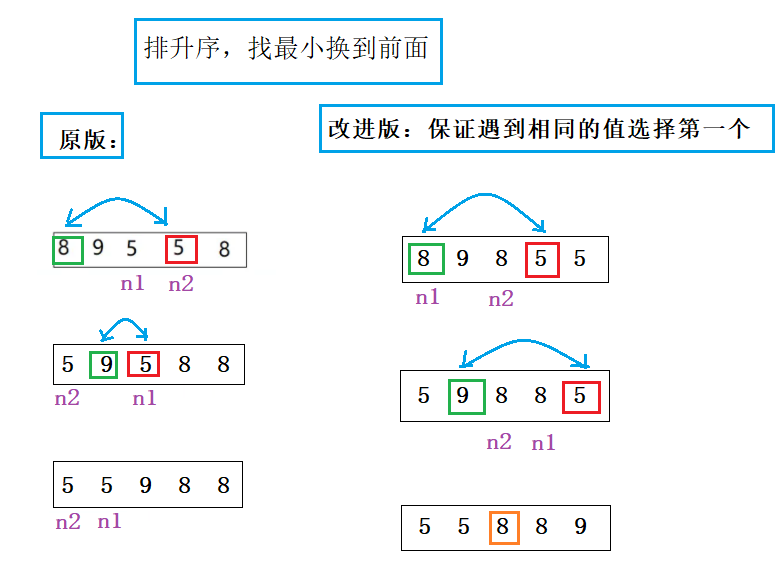#### 直接插入排序#### 希爾排序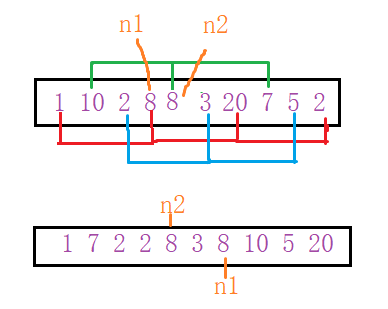#### 堆排序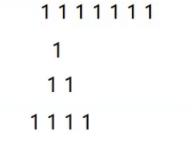#### 快速排序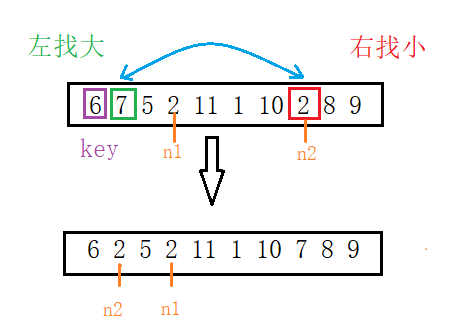### 排序需要掌握的知識點和程度

1. 幾大排序的原理和實現
2. 時間複雜度和空間複雜度（不要背，要靠理解）
3. 穩定性
4. 排序之間特性的對比「其他文章」